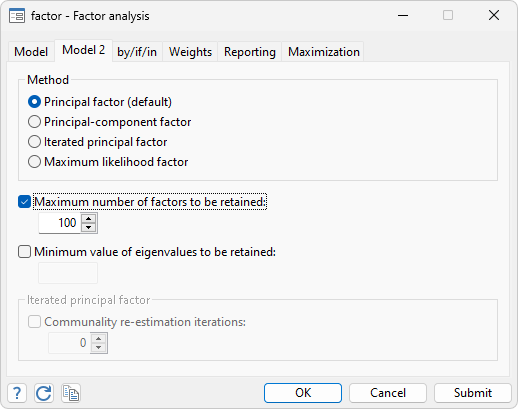Use multivariate analyses to evaluate relationships among variables from many different perspectives. Perform multivariate tests of means, or fit multivariate regression and MANOVA models. Explore relationships between two sets of variables, such as aptitude measurements and achievement measurements, using canonical correlation. Examine the number and structure of latent concepts underlying a set of variables using exploratory factor analysis. Or use principal component analysis to find underlying structure or to reduce the number of variables used in a subsequent analysis. Discover groupings of observations in your data using cluster analysis. If you have known groups in your data, describe differences between them using discriminant analysis. And much more.

FACTOR ANALYSIS

• Works on datasets or correlation matrices
• Principal-components factor
• Principal factor
• Iterated principal factor
• ML factors
• Rotations
• Orthogonal and oblique rotations
• Kaiser normalization
• Varimax, quartimax, oblimax, parsimax, equamax, and promax rotation
• Minimum entropy rotation
• Comrey’s tandem
• Rotate toward a target matrix
• Anti-image correlation matrices
• Kaiser–Meyer–Olkin measure of sampling adequacy
• Squared multiple correlations
• Bartlett scoring
• Regression scoringPRINCIPAL COMPONENTS

• Works with datasets or correlation or covariance matrices
• Standard errors of eigenvalues and vectors
• Anti-image correlation matrices
• Kaiser–Meyer–Olkin measure of sampling adequacy
• Squared multiple correlations
• Rotations
• Orthogonal and oblique rotations
• Kaiser normalization
• Varimax, quartimax, oblimax, parsimax, equamax, and promax rotation
• Minimum entropy rotation
• Comrey’s tandem
• Rotate toward a target matrix

DISCRIMINANT ANALYSIS

• Linear
• Logistic Updated
• kth nearest neighbor Updated
• Classification tables
• Error rates

ZELLNER’S SEEMINGLY UNRELATED REGRESSION

• Two-step or maximum likelihood estimates
• Linear constraints
• Breusch-Pagan test for independent equations

MULTIVARIATE LINEAR REGRESSION

• Breusch–Pagan test for independent equations
• Bayesian multivariate regression

PROCRUSTES ANALYSIS

• Orthogonal, oblique, and unrestricted transformations
• Overlaid graphs comparing target variables and fitted values of source variables

CANONICAL CORRELATIONS

• Correlation matrices
• Plot canonical correlations

TETRACHORIC CORRELATIONS

• Maximum likelihood or noniterative Edwards and Edwards estimator
• Tetrachoric correlation coefficient and standard error
• Exact two-sided significance level

STRUCTURAL EQUATION MODELING (SEM)

• Complete implementation

LATENT CLASS ANALYSIS

• Including latent profile analysis
• Including finite mixture models
• Marginal probabilities and marginal means
• Evaluate goodness of fit
• Predict probabilities of class membership and values of observed outcome variables

CLUSTER ANALYSIS

• Complete implementation

MANOVA

• Complete implementation

MULTIVARIATE TESTS

• One- and multisample
• Means, covariances, and correlations
• Tests of normality
• Doornik–Hansen
• Henze–Zirkler
• Two by Mardia

MULTIDIMENSIONAL SCALING

• Modern metric and nonmetric multidimensional scaling
• Classic metric multidimensional scaling
• Works with two-way data, proximity data in long format, and proximity data in a matrix
• 33 similarity/dissimilarity measures
• Coordinates of approximating configuration
• Correlations between dissimilarities and distances
• Kruskal stress measure
• Shepard diagram
• Plots of approximating Euclidean configuration

CORRESPONDENCE ANALYSIS

• Two-way correspondence analysis
• Work with cross-tabulations of categorical variables or matrices of counts
• Stacked (crossed) variables
• Fitted, observed, and expected correspondence tables
• Coordinates in column space
• Coordinates in row space (with two-way CA)
• Row and column profiles (conditional distributions)
• Chi-squared distances
• Correlations of profiles and axes
• Inertia contributions
• Biplots
• Projection plots
• Multiple and joint correspondence analysis (MCA and JCA)
• Work with cross-tabulations of categorical variables
• Stacked (crossed) variables
• Coordinates in column space
• Projection plots
• Matrix of inertias (after JCA)

POSTESTIMATION SELECTOR

• View and run all postestimation features for your command
• Automatically updated as estimation commands are run

BIPLOTS

• Display your choice of any two biplot dimensions
• Distinguish groups of data within the biplot
• Display table of biplot coordinates
• Generate new variables containing biplot coordinates

HOTELLING’S T-SQUARED

CRONBACH’S ALPHA

• Interitem correlations or covariances
• Generate summative scale
• Automatically reverse sense of variables